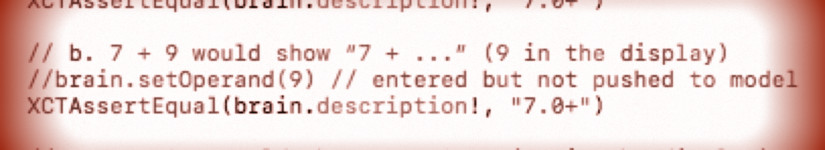# cs193p – Assignment #1 Task #6Add a String property to your CalculatorBrain called description which returns a description of the sequence of operands and operations that led to the value returned by result (or the result so far if resultIsPending). The character = (the equals sign) should never appear in this description, nor should … (ellipses).

First we need to change the accumulator into a tuple to hold also the description text:

```private var accumulator: (Double, String)?
```

Performing operations with constants, save the symbol of the constant as this description text:

```    mutating func performOperation(_ symbol: String) {
...
case .constant(let value):
accumulator = (value, symbol)
...
}
```

For the other operations we need to tell the calculator brain, how the descriptions of the various functions should look like. Unary operations this we use a function with a string parameter and a string return value:

```    private enum Operation {
...
case unaryOperation((Double) -> Double, (String) -> String)
...
}
```

Which look something like the following:

```    private let operations: Dictionary<String,Operation> = [
...
"√": Operation.unaryOperation(sqrt, { "√(" + \$0 + ")" }),
"cos": Operation.unaryOperation(cos, { "cos(" + \$0 + ")" }),
"±": Operation.unaryOperation({ -\$0 }, { "-(" + \$0 + ")" }),
...
"x²" : Operation.unaryOperation({ pow(\$0, 2) }, { "(" + \$0 + ")²" }),
"x³" : Operation.unaryOperation({ pow(\$0, 3) }, { "(" + \$0 + ")³" }),
"x⁻¹" : Operation.unaryOperation({ 1 / \$0 }, {  "(" + \$0 + ")⁻¹" }),
"sin" : Operation.unaryOperation(sin, { "sin(" + \$0 + ")" }),
"tan" : Operation.unaryOperation(tan, { "tan(" + \$0 + ")" }),
"sinh" : Operation.unaryOperation(sinh, { "sinh(" + \$0 + ")" }),
"cosh" : Operation.unaryOperation(cosh, { "cosh(" + \$0 + ")" }),
"tanh" : Operation.unaryOperation(tanh, { "tanh(" + \$0 + ")" }),
"ln" : Operation.unaryOperation(log, { "ln(" + \$0 + ")" }),
"log" : Operation.unaryOperation(log10, { "log(" + \$0 + ")" }),
"eˣ" : Operation.unaryOperation(exp, { "e^(" + \$0 + ")" }),
"10ˣ" : Operation.unaryOperation({ pow(10, \$0) }, { "10^(" + \$0 + ")" }),
"x!" : Operation.unaryOperation(factorial, { "(" + \$0 + ")!" }),
...
]
```

Use this new description functions to fill the description part of the acumulator – and because the accumulator part is no simple Double any more, adjust the value part as well:

```    mutating func performOperation(_ symbol: String) {
...
case .unaryOperation(let function, let description):
if nil != accumulator {
accumulator = (function(accumulator!.0), description(accumulator!.1))
}
...
}
```

Do the same for binary operations, create a possibility to provide the description function (now with tow parameters):

```    private enum Operation {
...
case binaryOperation((Double, Double) -> Double, (String, String) -> String)
...
}
```

Provide those functions:

```    private let operations: Dictionary<String,Operation> = [
...
"×": Operation.binaryOperation(*, { \$0 + "×" + \$1 }),
"÷": Operation.binaryOperation(/, { \$0 + "÷" + \$1 }),
"+": Operation.binaryOperation(+, { \$0 + "+" + \$1 }),
"-": Operation.binaryOperation(-, { \$0 + "-" + \$1 }),
"=": Operation.equals,
...
"xʸ" : Operation.binaryOperation(pow, { \$0 + "^" + \$1 }),
]
```

And use them performing operations:

```    mutating func performOperation(_ symbol: String) {
...
case .binaryOperation(let function, let description):
performPendingBinaryOperation()
if nil != accumulator {
pendingBinaryOperation = PendingBinaryOperation(function: function, description: description, firstOperand: accumulator!)
accumulator = nil
}
...
}
```

To allow the above code to work we need to adjust pending-binary-operation structure. Add a new property for the description function, and allow the operand to cope with the new accumulator type. Finally use them performing pending binary operations:

```    private struct PendingBinaryOperation {
...
let description: (String, String) -> String
let firstOperand: (Double, String)

func perform(with secondOperand: (Double, String)) -> (Double, String) {
return (function(firstOperand.0, secondOperand.0), description(firstOperand.1, secondOperand.1))
}
}
```

For operands, just use the “Double” value as string description:

```    mutating func setOperand(_ operand: Double) {
accumulator = (operand, "\(operand)")
}
```

Because the accumulator is no simple Optional any more, we need also to change the result property:

```    var result: Double? {
get {
if nil != accumulator {
return accumulator!.0
}
return nil
}
}
```

… now really finally, add the requested description property. If there is a result pending and we have a valid accumulator, show them. Otherwise only the current data for the pending operation, or just return the accumulator, if there is any:

```    var description: String? {
get {
if resultIsPending {
return pendingBinaryOperation!.description(pendingBinaryOperation!.firstOperand.1, accumulator?.1 ?? "")
} else {
return accumulator?.1
}
}
}
```

The code so far provides no output of the description property. For testing add a debugging output somewhere or some unit tests (like I did, have a look at the code).

This implementation of the description is actually faulty. It shows e.g. “1.0+2.0*3.0” which would result in “7.0”. However the calculator gets a result of “9.0” which should match a description of “(1.0+2.0)*3.0”. Feeling lazy, I will skip adding brackets to the description because the assignment does not ask for it and we solved that problem already in the assignments of the previous years.

The complete code for the assignment #1 task #6 is available on GitHub.

## 3 thoughts on “cs193p – Assignment #1 Task #6”

1. RR says:

Hi, thanks for posting this, I am following along with iTunesU so do not have access to much help when I get stuck. Question: I noticed that in your code, you tend to use

if nil != accumulator {

Why not “if accumulator != nil {” ? Is this just a stylistic difference, or is there another benefit to flipping the order?

1. Abuzar Manzoor says:

how does the description work
this code
“let description: (String, String) -> String”
can you plz provide me the hint, i am not getting it.